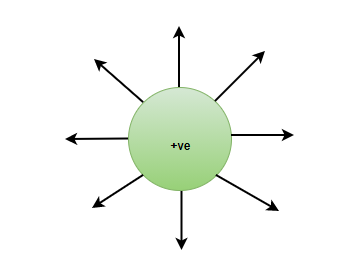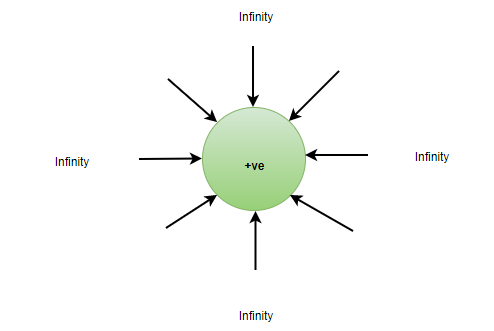Related Articles

# Basic Properties of Electric Charge

• Last Updated : 24 Jun, 2021

The presence of electric charges are not only seen in the field of science but also in the daily lives of human beings, for instance, rubbing the dry hair with a ruler ends up making some hair strands stand up and this happens because electric charges are present everywhere in everything. The example just mentioned was the example of electric charges temporarily getting charged.

### Electric Charge

It is the basic physical property of matter and due to this property, a force is experienced when kept in the field of electricity. Electric charges are of two types,

• Positive Charge

Positive charges or protons have a charge of +1.6 × 10-19 Coulomb. A positive charge has its field lines emerging from within and going upto infinity.• Negative Charge

Negative charges or Electrons have a charge of -1.6 × 10-19 Coulomb. A Negative charge has its field lines coming from infinity.The above example where hair strands start to attract to the ruler is due to electric charges. Similarly, rubbing a balloon on hair attracts hair to the balloon, in case two balloons are simultaneously rubbed, the balloons will start to repel each other, but they will attract the hair strands.

The rate of change of electric charge is known as Electric current,

I = q/t

### Properties of Electric Charges

In order to look at the properties of electric charge, consider the electric charges to be really small, known as the Point charge. Point charges are smaller than the distance between them.

Electric charges when they are considered as point charges are scalar in nature. With that, it is important to note that charges can be point charges, but they are still positive and negative charges. The additive property of electric charges says that if there are n number of charges present inside, The total charge present will be the algebraic sum of the individual charges.

Q = q1+ q2+ q3+….. qn

• Conservation of Charges

The Conservation of charges says that the charges are neither created not destroyed. They can be transferred from one body to another, but they cannot be created or destroyed. In an Isolated system, the charges are always conserved.

• Quantization of Charge

According to the quantization of electric charge, Electric charges are defined as the Integral multiple of the charge present on them, hence, in any system, The charges will be,

q = ne

Where,

n = Integer numbers

e = value of the charge (1.6× 10-19 C)

Some other properties of electric charge

• Charge is a scalar quantity.
• Charge is transferrable, they transfer from one body to another.
• Like charges repel each other and unlike charges attract each other.
• Charge is always associated with mass.

### Sample Questions

Question 1: What are three basic properties of Charge?

The three basic properties of Charge:

2. Quantization of charges
3. Conservation of charge

Question 2: A polythene is rubbed against a woolen cloth, the charge developed on the woolen cloth is 7× 10-9C. What is the amount of charges transferred ?

Solution:

The Total charge present = 7 × 10-9 C.

q= 1.6 10-19 C.

Q = ne

7× 10-9= n × 1.6 × 10-19

n = 4.375 × 1010 C.

Question 3: Can Charge Exist without Mass?

One of the basic properties of electric charge is that the Charge is always associated Mass. A charge does not exist without mass.

Question 4: How many electrons are present in one Coulomb?

Solution:

Charge on 1 Electrons = 1.6× 10-19C

Number of electrons present in 1 Coulomb = 1/(1.6× 10-19)

= 6.25 × 1018 Electrons.

Question 5: 5 different types of charges are present in an isolated system, the values of the charges are- +5nC, -6nC, +3nC, +4nC, +1nC. What is the total charge present in the system?

Solution:

Additivity property of electric charges is,

Q = q1+ q2+ q3+ q4+ q5

Q = (+5 – 6+ 3+ 4+ 1)nC

Q = 7nC

Question 6: If a system has 0 charge overall. Is it true that there are no charges present in the system?